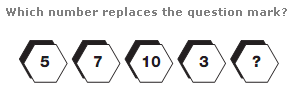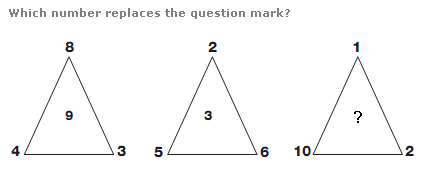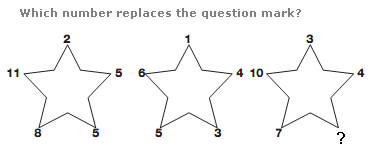# Puzzles - Number puzzles

### Exercise :: Number puzzlesAnswer : 5 Explanation : Take pairs of numbers at opposite ends of the diagram. Their total is always 10.Answer : 11 Explanation : In each triangle, the central value equals the sum of the 2 even numbers from the outside corners of the triangle minus the odd number.Answer : 5 Explanation : Start at the top of each triangle and move clockwise around its points. Add the first two numbers together and subtract 2 to give the next value round.